# Seurat进行单细胞RNA-seq数据整合

• 标准流程

```setwd('/your/path/to/seurat')
#加载所需要的包
library(Seurat)
library(SeuratData)
#数据已经整合到SeuratData包中
#InstallData("panc8")
#将数据按技术进行拆分
data("panc8")
pancreas.list <- SplitObject(panc8, split.by = "tech")
pancreas.list <- pancreas.list[c("celseq", "celseq2", "fluidigmc1", "smartseq2")]
```

```#在寻找anchor之前先进行标准化，找出各个数据集自身的差异表达基因
for (i in 1:length(pancreas.list)) {
pancreas.list[[i]] <- NormalizeData(pancreas.list[[i]], verbose = FALSE)
pancreas.list[[i]] <- FindVariableFeatures(pancreas.list[[i]], selection.method = "vst",
nfeatures = 2000, verbose = FALSE)
}
```

```#寻找anchor，这里只用了其中的三个数据，另一个数据会在后面用到
reference.list <- pancreas.list[c("celseq", "celseq2", "smartseq2")]
pancreas.anchors <- FindIntegrationAnchors(object.list = reference.list, anchor.features = 2000,dims = 1:30)```

```#利用这样的anchor去矫正批次和技术差异（相当于找到了共同的锚点，用这样的锚点去矫正表达水平）
pancreas.integrated <- IntegrateData(anchorset = pancreas.anchors, dims = 1:30)
```

```#将seurat对象的默认assay设置成整合后的表达矩阵
DefaultAssay(pancreas.integrated) <- "integrated"
```

```#载入包
library(ggplot2)
library(cowplot)
#归一化，注意，这里只有2000个基因
pancreas.integrated <- ScaleData(pancreas.integrated, verbose = FALSE)
```

```#PCA
pancreas.integrated <- RunPCA(pancreas.integrated, npcs = 30, verbose = FALSE)
```

```#使用umap根据pca降维的情况，取其前30个（总共也就30个）PC作为降维的高维向量
pancreas.integrated <- RunUMAP(pancreas.integrated, reduction = "pca", dims = 1:30)
#可视化，分别以技术和细胞类型作为标签
p1 <- DimPlot(pancreas.integrated, reduction = "umap", group.by = "tech")
p2 <- DimPlot(pancreas.integrated, reduction = "umap", group.by = "celltype", label = TRUE,
repel = TRUE)   NoLegend()
plot_grid(p1, p2)
```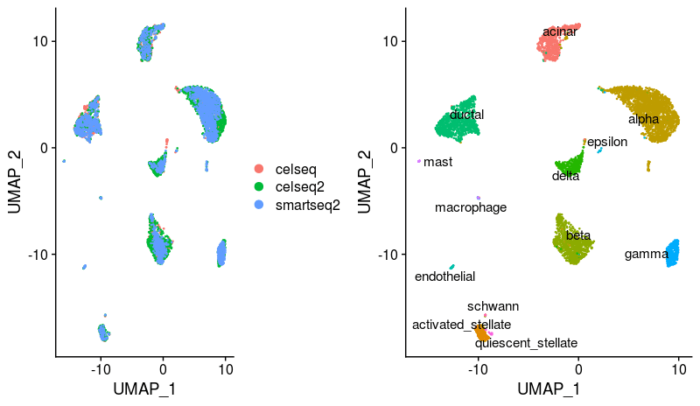```#类似的，也可以基于anchor实现分类器（label transfer）
#需要注意的是，与整合不同，分类器只是预测每个细胞在参考数据中的细胞类型，并不会对表达矩阵进行修正
#这里使用之前还未使用的另一组数据
pancreas.query <- pancreas.list[["fluidigmc1"]]
pancreas.anchors <- FindTransferAnchors(reference = pancreas.integrated, query = pancreas.query,
dims = 1:30)
#这里寻找transfer anchor有两种方法，一种是基于PCA的方法，一种是基于CCA的方法，默认且官方推荐PCA的方法
```

```#TransferData函数作为分类机，可以基于anchor的模型进行分类，得到预测结果
predictions <- TransferData(anchorset = pancreas.anchors, refdata = pancreas.integrated\$celltype,
dims = 1:30)
```

```#查看预测准确率
prediction.match <- pancreas.query\$predicted.id == pancreas.query\$celltype
table(prediction.match)
```

```> table(prediction.match)
prediction.match
FALSE  TRUE
18   620
```

```> table(pancreas.query\$predicted.id)
acinar activated_stellate              alpha               beta
21                 17                248                258
delta             ductal        endothelial            epsilon
22                 33                 13                  1
gamma         macrophage               mast            schwann
17                  1                  2                  5
```

```#进一步通过marker基因的表达来判断预测的准确性
VlnPlot(pancreas.query, c("REG1A", "PPY", "SST", "GHRL", "VWF", "SOX10"), group.by = "predicted.id")
```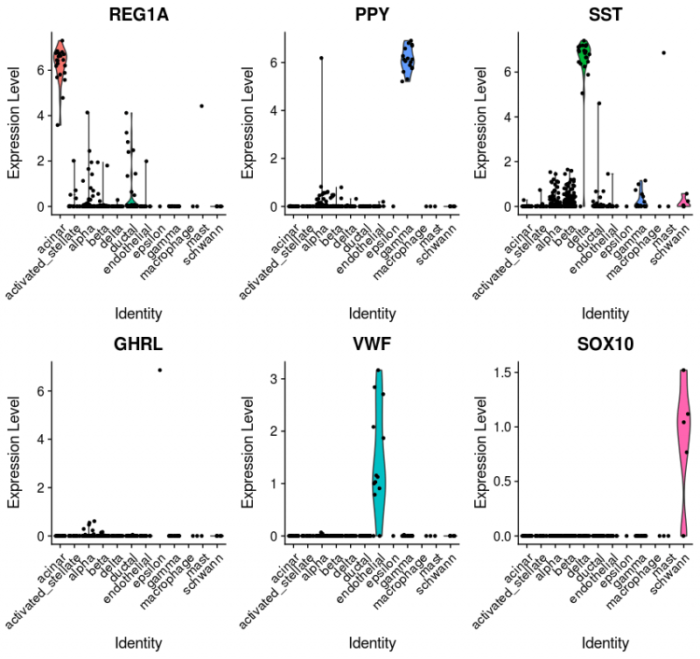```setwd('/your/path/to/seurat')
#加载所需要的包
library(Seurat)
library(SeuratData)
#先尝试标准工作流程，后面尝试其他的高级姿势
#标准工作流程，可以实现两个目标
#1.将多个不同的单细胞RNA-seq数据整合成一个大的reference
#2.作为分类器，将一个已经定义好的参考数据库中的细胞标签，转移到一个新的数据集中，相当于用参考数据库对新数据中的cell类型进行分类
#数据已经整合到SeuratData包中
#标准流程是将使用四种不同测序技术得到的人类胰岛细胞数据整合起来
#InstallData("panc8")
#将数据按技术进行拆分
data("panc8")
pancreas.list <- SplitObject(panc8, split.by = "tech")
pancreas.list <- pancreas.list[c("celseq", "celseq2", "fluidigmc1", "smartseq2")]
#来看下数据结构，pancrease.list包含了4个seurat对象
#流程
#在寻找anchor之前先进行标准化，找出各个数据集自身的差异表达基因
for (i in 1:length(pancreas.list)) {
pancreas.list[[i]] <- NormalizeData(pancreas.list[[i]], verbose = FALSE)
pancreas.list[[i]] <- FindVariableFeatures(pancreas.list[[i]], selection.method = "vst",
nfeatures = 2000, verbose = FALSE)
}
#寻找anchor，这里只用了其中的三个数据，另一个数据会在后面用到
reference.list <- pancreas.list[c("celseq", "celseq2", "smartseq2")]
pancreas.anchors <- FindIntegrationAnchors(object.list = reference.list, anchor.features = 2000, dims = 1:30)
#dims取了默认参数，也可以进行调整，官网推荐值在10-50之间
#利用这样的anchor去矫正批次和技术差异（相当于找到了共同的锚点，用这样的锚点去矫正表达水平）
pancreas.integrated <- IntegrateData(anchorset = pancreas.anchors, dims = 1:30)
#看一下数据结构，IntegrateData这个函数可以将锚点信息转换成整合的表达矩阵，在assay中可以看到，除了整合之前的表达矩阵存储在RNA中，还多了整合后的表达矩阵存储在integrated中
#这两个表达矩阵可以通过DefaultAssay函数进行切换
#另外值得注意的是，在整合后的表达矩阵中，只有2000行，对应了所找到的差异表达基因
#这个新的整合后的表达矩阵就可以进行一系列的下游分析了
#老规矩，按细胞类型（非技术类型）进行聚类，再用tSNE或者UMAP可视化
library(ggplot2)
library(cowplot)
#将seurat对象的默认assay设置成整合后的表达矩阵
DefaultAssay(pancreas.integrated) <- "integrated"
#归一化，注意，这里只有2000个基因
pancreas.integrated <- ScaleData(pancreas.integrated, verbose = FALSE)
#PCA
pancreas.integrated <- RunPCA(pancreas.integrated, npcs = 30, verbose = FALSE)
#使用umap根据pca降维的情况，取其前30个（总共也就30个）PC作为降维的高维向量
pancreas.integrated <- RunUMAP(pancreas.integrated, reduction = "pca", dims = 1:30)
#可视化，分别以技术和细胞类型作为标签
p1 <- DimPlot(pancreas.integrated, reduction = "umap", group.by = "tech")
p2 <- DimPlot(pancreas.integrated, reduction = "umap", group.by = "celltype", label = TRUE,
repel = TRUE)   NoLegend()
plot_grid(p1, p2)
#类似的，也可以基于anchor实现分类器（label transfer）
#需要注意的是，与整合不同，分类器只是预测每个细胞在参考数据中的细胞类型，并不会对表达矩阵进行修正
#这里使用之前还未使用的另一组数据
pancreas.query <- pancreas.list[["fluidigmc1"]]
pancreas.anchors <- FindTransferAnchors(reference = pancreas.integrated, query = pancreas.query,
dims = 1:30)
#这里寻找transfer anchor有两种方法，一种是基于PCA的方法，一种是基于CCA的方法，默认且官方推荐PCA的方法
#TransferData函数作为分类机，可以基于anchor的模型进行分类，得到预测结果
predictions <- TransferData(anchorset = pancreas.anchors, refdata = pancreas.integrated\$celltype,
dims = 1:30)
#查看预测准确率
prediction.match <- pancreas.query\$predicted.id == pancreas.query\$celltype
table(prediction.match)
#准确率97.18%
table(pancreas.query\$predicted.id)
#根据官方的说法，即使测试集中只有少数几个细胞代表某种细胞类型，可以用这种方法进行预测，比如epsilon
#进一步通过marker基因的表达来判断预测的准确性
VlnPlot(pancreas.query, c("REG1A", "PPY", "SST", "GHRL", "VWF", "SOX10"), group.by = "predicted.id")
```
• SC Transform

SC Transform相当于是标准流程的一种modified版本，其原理和标准流程类似，只不过标准流程中使用log函数对表达矩阵进行标准化，这里使用SC transform对表达矩阵进行标准化，再对皮尔逊残差进行协调整合。直接上代码：

```setwd('/your/path/to/seurat')
#原理和标准流程是类似的，只不过标准流程中使用log函数对表达矩阵进行标准化，这里使用SC transform对表达矩阵进行标准化，再对皮尔逊残差进行协调整合
#加载包
library(Seurat)
library(ggplot2)
library(SeuratData)
#可能是数据比较大吧，他这里设置了一下全局变量的最大容量
options(future.globals.maxSize = 4000 * 1024^2)
#还是之前的4组数据
data("panc8")
pancreas.list <- SplitObject(panc8, split.by = "tech")
pancreas.list <- pancreas.list[c("celseq", "celseq2", "fluidigmc1", "smartseq2")]
```

```#第一步肯定还是标准化，在标准流程中用了NormalizeData函数，默认取logNormalize，这里使用ST transform进行标准化
for (i in 1:length(pancreas.list)) {
pancreas.list[[i]] <- SCTransform(pancreas.list[[i]], verbose = T)
}
#因为用时较长，建议把verbose设置成True
```

```#选择用于下游寻找anchor并整合的feature，这里选择3000个基因
pancreas.features <- SelectIntegrationFeatures(object.list = pancreas.list, nfeatures = 3000)
```

```#整合前预处理，保证所有必要的皮尔逊残差都被计算到
pancreas.list <- PrepSCTIntegration(object.list = pancreas.list, anchor.features = pancreas.features, verbose = T)
```

```#整合步骤与标准流程类似，不过需要注意标准化方法选择SCT
pancreas.anchors <- FindIntegrationAnchors(object.list = pancreas.list, normalization.method = "SCT",
anchor.features = pancreas.features, verbose = T)
pancreas.integrated <- IntegrateData(anchorset = pancreas.anchors, normalization.method = "SCT",
verbose = T)
```

```#那么和标准流程一样的，下面就可以进行各种下游分析了
#有一个注意点是SCT整合后就不要用ScaleData进行scale了
DefaultAssay(pancreas.integrated) <- "integrated"
pancreas.integrated <- RunPCA(pancreas.integrated, verbose = FALSE)
pancreas.integrated <- RunUMAP(pancreas.integrated, dims = 1:30)
plots <- DimPlot(pancreas.integrated, group.by = c("tech", "celltype"), combine = FALSE)
plots <- lapply(X = plots, FUN = function(x) x   theme(legend.position = "top")   guides(color = guide_legend(nrow = 3, byrow = TRUE, override.aes = list(size = 3))))
CombinePlots(plots)
#这画图操作可真骚
```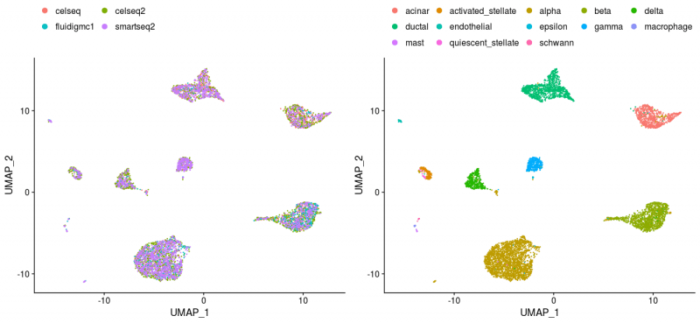```setwd('/your/path/to/seurat')
#原理和标准流程是类似的，只不过标准流程中使用log函数对表达矩阵进行标准化，这里使用ST transform对表达矩阵进行标准化，再对皮尔逊残差进行协调整合
#加载包
library(Seurat)
library(ggplot2)
library(SeuratData)
#可能是数据比较大吧，他这里设置了一下全局变量的最大容量
options(future.globals.maxSize = 4000 * 1024^2)
#还是之前的4组数据
data("panc8")
pancreas.list <- SplitObject(panc8, split.by = "tech")
pancreas.list <- pancreas.list[c("celseq", "celseq2", "fluidigmc1", "smartseq2")]
#第一步肯定还是标准化，在标准流程中用了NormalizeData函数，默认取logNormalize，这里使用ST transform进行标准化
for (i in 1:length(pancreas.list)) {
pancreas.list[[i]] <- SCTransform(pancreas.list[[i]], verbose = T)
}
#因为用时较长，建议把verbose设置成True
#选择用于下游寻找anchor并整合的feature，这里选择3000个基因
pancreas.features <- SelectIntegrationFeatures(object.list = pancreas.list, nfeatures = 3000)
#整合前预处理，保证所有必要的皮尔逊残差都被计算到
pancreas.list <- PrepSCTIntegration(object.list = pancreas.list, anchor.features = pancreas.features, verbose = T)
#整合步骤与标准流程类似，不过需要注意标准化方法选择SCT
pancreas.anchors <- FindIntegrationAnchors(object.list = pancreas.list, normalization.method = "SCT",
anchor.features = pancreas.features, verbose = T)
pancreas.integrated <- IntegrateData(anchorset = pancreas.anchors, normalization.method = "SCT",
verbose = T)
#那么和标准流程一样的，下面就可以进行各种下游分析了
#有一个注意点是SCT整合后就不要用ScaleData进行scale了
DefaultAssay(pancreas.integrated) <- "integrated"
pancreas.integrated <- RunPCA(pancreas.integrated, verbose = FALSE)
pancreas.integrated <- RunUMAP(pancreas.integrated, dims = 1:30)
plots <- DimPlot(pancreas.integrated, group.by = c("tech", "celltype"), combine = FALSE)
plots <- lapply(X = plots, FUN = function(x) x   theme(legend.position = "top")   guides(color = guide_legend(nrow = 3, byrow = TRUE, override.aes = list(size = 3))))
CombinePlots(plots)
#这画图操作可真骚
#可以看到，大部分细胞也都按照各自的label与其他细胞类群区分了开来，说明合并是有效的
```
• Reference-based

Reference-based integration也是标准流程的一种modified版本，在之前的分析中，无论是标准流程还是SC Transform，在寻找anchor的过程中都要在数据集之间进行两两比较，每个数据集在下游分析中都有相同的权重，因此其计算开销是非常大的，在上面的SC Transform中我也有所感触。那么reference-based integration的想法就是，把其中的一个或几个数据集设置成reference，那么在寻找anchor的时候就不需要将所有的数据集两两比较了，而只需要将query数据集和reference数据集一一比较即可，从而大大减小了计算量。比如我有10个数据集，那么两两比较需要计算45次，但如果把一个数据集设置成reference，则只需要将剩下的9个数据集和reference数据集比较9次即可。因此，这种reference-based integration适用于数据集较多的情况下的整合，并且对于log标准化还是SC Transform标准化也都是适用的。

```setwd('/your/path/to/seurat')
#reference-based integration和标准流程也是类似的，其区别在于可以将其中的一个或几个数据集设置成reference，从而减少计算量
#数据使用的是用8种技术制备的人类PBMC数据集，可以在SeuratData包中找到
#载入包
library(Seurat)
library(SeuratData)
library(ggplot2)
#载入人类PBMC数据集
data("pbmcsca")
pbmc.list <- SplitObject(pbmcsca, split.by = "Method")
```

```#标准化，使用SC Transform
for (i in names(pbmc.list)) {
pbmc.list[[i]] <- SCTransform(pbmc.list[[i]], verbose = T)
}
```

```#选择用于寻找anchor和下游分析的feature，这里仍然选择3000个基因
#挑选完基因后，即可对相应的皮尔逊残差进行计算，完成整合前的预处理
pbmc.features <- SelectIntegrationFeatures(object.list = pbmc.list, nfeatures = 3000)
pbmc.list <- PrepSCTIntegration(object.list = pbmc.list, anchor.features = pbmc.features)
```

```#选择参考数据集
reference_dataset <- which(names(pbmc.list) == "10x Chromium (v3)")
#这里的返回值是5，如果想设置几个数据集为一个reference，可以使用c(5,6)等类似的写法
```

```#计算anchor并整合，与SC Transform唯一的区别在于多了reference参数
pbmc.anchors <- FindIntegrationAnchors(object.list = pbmc.list, normalization.method = "SCT", anchor.features = pbmc.features, reference = reference_dataset)
pbmc.integrated <- IntegrateData(anchorset = pbmc.anchors, normalization.method = "SCT")
```

```#可视化
DefaultAssay(pancreas.integrated) <- "integrated"
pbmc.integrated <- RunPCA(object = pbmc.integrated, verbose = T)
pbmc.integrated <- RunUMAP(object = pbmc.integrated, dims = 1:30)
plots <- DimPlot(pbmc.integrated, group.by = c("Method", "CellType"), combine = FALSE)
plots <- lapply(X = plots, FUN = function(x) x   theme(legend.position = "top")   guides(color = guide_legend(nrow = 4, byrow = TRUE, override.aes = list(size = 2.5))))
CombinePlots(plots)```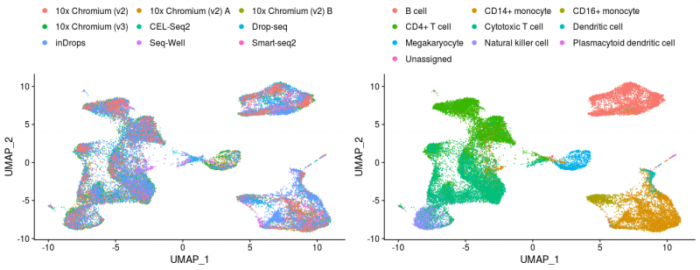```#按照技术将图片拆分
DimPlot(pbmc.integrated, group.by = "CellType", split.by = "Method", ncol = 3)
```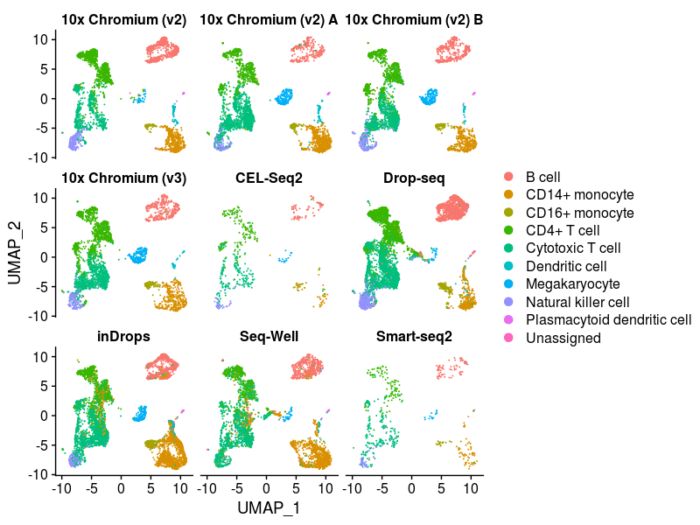```#使用maker基因的表达量进一步验证聚类情况
DefaultAssay(pbmc.integrated) <- "RNA"
#对RNA中存储的原始表达矩阵进行标准化
pbmc.integrated <- NormalizeData(pbmc.integrated, verbose = T)
FeaturePlot(pbmc.integrated, c("CCR7", "S100A4", "GZMB", "GZMK", "GZMH", "TCL1A"))
#FeaturePlot也可以用method进行split
```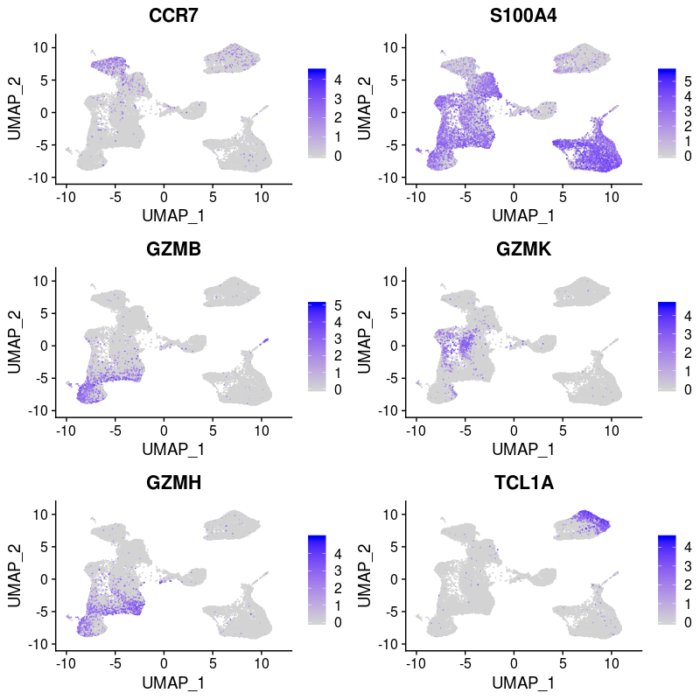```setwd('/your/path/to/seurat')
#reference-based integration和标准流程也是类似的，其区别在于可以将其中的一个或几个数据集设置成reference，从而减少计算量
#数据使用的是用8种技术制备的人类PBMC数据集，可以在SeuratData包中找到
#载入包
library(Seurat)
library(SeuratData)
library(ggplot2)
#载入人类PBMC数据集
data("pbmcsca")
pbmc.list <- SplitObject(pbmcsca, split.by = "Method")
#标准化，使用SC Transform
for (i in names(pbmc.list)) {
pbmc.list[[i]] <- SCTransform(pbmc.list[[i]], verbose = T)
}
#选择用于寻找anchor和下游分析的feature，这里仍然选择3000个基因
#挑选完基因后，即可对相应的皮尔逊残差进行计算，完成整合前的预处理
pbmc.features <- SelectIntegrationFeatures(object.list = pbmc.list, nfeatures = 3000)
pbmc.list <- PrepSCTIntegration(object.list = pbmc.list, anchor.features = pbmc.features)
#选择参考数据集
reference_dataset <- which(names(pbmc.list) == "10x Chromium (v3)")
#这里的返回值是5，如果想设置几个数据集为一个reference，可以使用c(5,6)等类似的写法
#计算anchor并整合，与SC Transform唯一的区别在于多了reference参数
pbmc.anchors <- FindIntegrationAnchors(object.list = pbmc.list, normalization.method = "SCT",
anchor.features = pbmc.features, reference = reference_dataset)
pbmc.integrated <- IntegrateData(anchorset = pbmc.anchors, normalization.method = "SCT")
#可视化
DefaultAssay(pancreas.integrated) <- "integrated"
pbmc.integrated <- RunPCA(object = pbmc.integrated, verbose = T)
pbmc.integrated <- RunUMAP(object = pbmc.integrated, dims = 1:30)
plots <- DimPlot(pbmc.integrated, group.by = c("Method", "CellType"), combine = FALSE)
plots <- lapply(X = plots, FUN = function(x) x   theme(legend.position = "top")   guides(color = guide_legend(nrow = 4,
byrow = TRUE, override.aes = list(size = 2.5))))
CombinePlots(plots)
#按照技术将图片拆分
DimPlot(pbmc.integrated, group.by = "CellType", split.by = "Method", ncol = 3)
#使用maker基因的表达量进一步验证聚类情况
DefaultAssay(pbmc.integrated) <- "RNA"
#对RNA中存储的原始表达矩阵进行标准化
pbmc.integrated <- NormalizeData(pbmc.integrated, verbose = T)
FeaturePlot(pbmc.integrated, c("CCR7", "S100A4", "GZMB", "GZMK", "GZMH", "TCL1A"))
#FeaturePlot也可以用method进行split
```
• Reciprocal PCA

```setwd('/your/path/to/seurat')
#使用reciprocal PCA的方法寻找数据集之间的anchor，从而进行整合
#数据使用SeuratData提供的人类免疫细胞图谱的数据集的downsample，共40k个细胞
#没有用完整数据是因为我根据提示找不到原始数据，我好菜
#加载包
library(Seurat)
library(SeuratData)
library(ggplot2)
#用于并行计算的包
library(future)
library(future.apply)
#初始化设置
plan("multiprocess", workers = 4)
options(future.globals.maxSize = 20000 * 1024^2)
#加载数据
# AvailableData()
# InstallData("hcabm40k.SeuratData")
data(hcabm40k)
```

```#将数据按来源拆分，并行计算标准化并寻找差异表达基因各2000个
bm40k.list <- SplitObject(hcabm40k, split.by = "orig.ident")
bm40k.list <- lapply(X = bm40k.list, FUN = function(x) {
x <- NormalizeData(x, verbose = FALSE)
x <- FindVariableFeatures(x, verbose = FALSE)
})
#我猜测在这里运行FindVariableFeatures是为了节省下面scale步骤中的时间，因为scale默认只scale差异表达基因
```

```#挑选用于整合的基因
features <- SelectIntegrationFeatures(object.list = bm40k.list)
```

Reciprocal PCA整合前，先对每个数据集进行PCA主成分分析，注意在跑PCA之前要先scale。

```#rPCA整合前，先scale再做PCA
bm40k.list <- lapply(X = bm40k.list, FUN = function(x) {
x <- ScaleData(x, features = features, verbose = FALSE)
x <- RunPCA(x, features = features, verbose = FALSE)
})
```

```#使用一个男人和一个女人的数据作为reference进行整合，注意降维选择rpca
anchors <- FindIntegrationAnchors(object.list = bm40k.list, reference = c(1, 2), reduction = "rpca", dims = 1:30)
bm40k.integrated <- IntegrateData(anchorset = anchors, dims = 1:30)
```

```#下游分析与可视化
bm40k.integrated <- ScaleData(bm40k.integrated, verbose = T)
bm40k.integrated <- RunPCA(bm40k.integrated, verbose = T)
bm40k.integrated <- RunUMAP(bm40k.integrated, dims = 1:30)
DimPlot(bm40k.integrated, group.by = "orig.ident")
```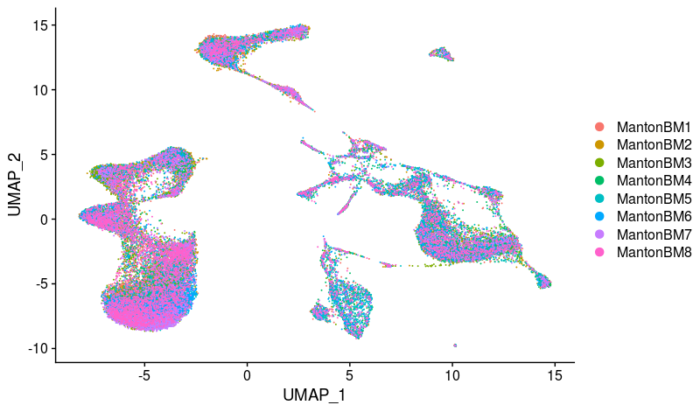```setwd('/your/path/to/seurat')
#使用reciprocal PCA的方法寻找数据集之间的anchor，从而进行整合
#数据使用SeuratData提供的人类免疫细胞图谱的数据集的downsample，共40k个细胞
#没有用完整数据是因为我根据提示找不到原始数据，我好菜
#加载包
library(Seurat)
library(SeuratData)
library(ggplot2)
#用于并行计算的包
library(future)
library(future.apply)
#初始化设置
plan("multiprocess", workers = 4)
options(future.globals.maxSize = 20000 * 1024^2)
#加载数据
# AvailableData()
# InstallData("hcabm40k.SeuratData")
data(hcabm40k)
#将数据按来源拆分，并行计算标准化并寻找差异表达基因各2000个
bm40k.list <- SplitObject(hcabm40k, split.by = "orig.ident")
bm40k.list <- lapply(X = bm40k.list, FUN = function(x) {
x <- NormalizeData(x, verbose = FALSE)
x <- FindVariableFeatures(x, verbose = FALSE)
})
#我猜测在这里运行FindVariableFeatures是为了节省下面scale步骤中的时间，因为scale默认只scale差异表达基因
#挑选用于整合的基因
features <- SelectIntegrationFeatures(object.list = bm40k.list)
#rPCA整合前，先scale再做PCA
bm40k.list <- lapply(X = bm40k.list, FUN = function(x) {
x <- ScaleData(x, features = features, verbose = FALSE)
x <- RunPCA(x, features = features, verbose = FALSE)
})
#使用一个男人和一个女人的数据作为reference进行整合，注意降维选择rpca
anchors <- FindIntegrationAnchors(object.list = bm40k.list, reference = c(1, 2), reduction = "rpca", dims = 1:30)
bm40k.integrated <- IntegrateData(anchorset = anchors, dims = 1:30)
#下游分析与可视化
bm40k.integrated <- ScaleData(bm40k.integrated, verbose = T)
bm40k.integrated <- RunPCA(bm40k.integrated, verbose = T)
bm40k.integrated <- RunUMAP(bm40k.integrated, dims = 1:30)
DimPlot(bm40k.integrated, group.by = "orig.ident")
```

OK，那么使用seurat进行单细胞RNA-seq数据整合的教程就到这里结束了。

• 版权声明 本文源自 傻狗的BLOG！ 整理 发表
• 转载请务必保留本文链接：https://www.plob.org/article/24435.html